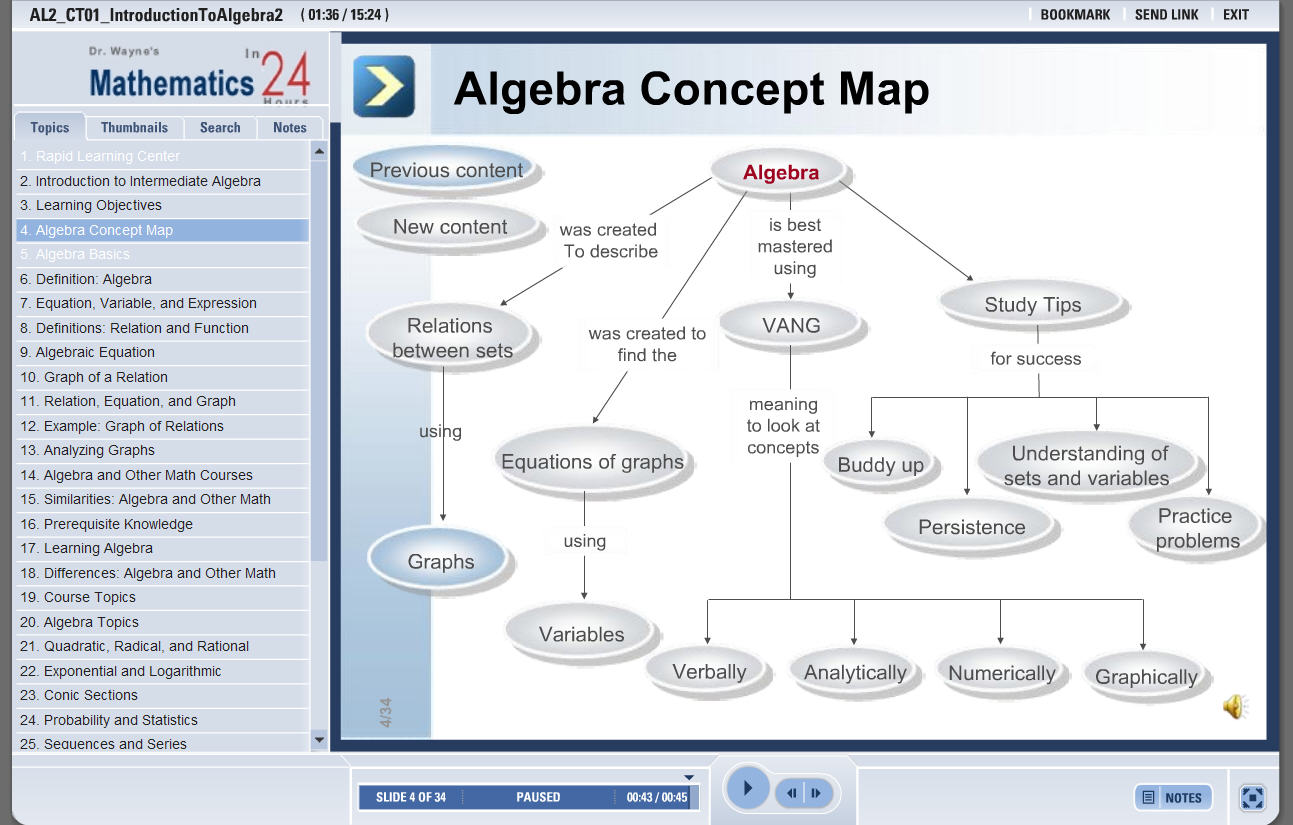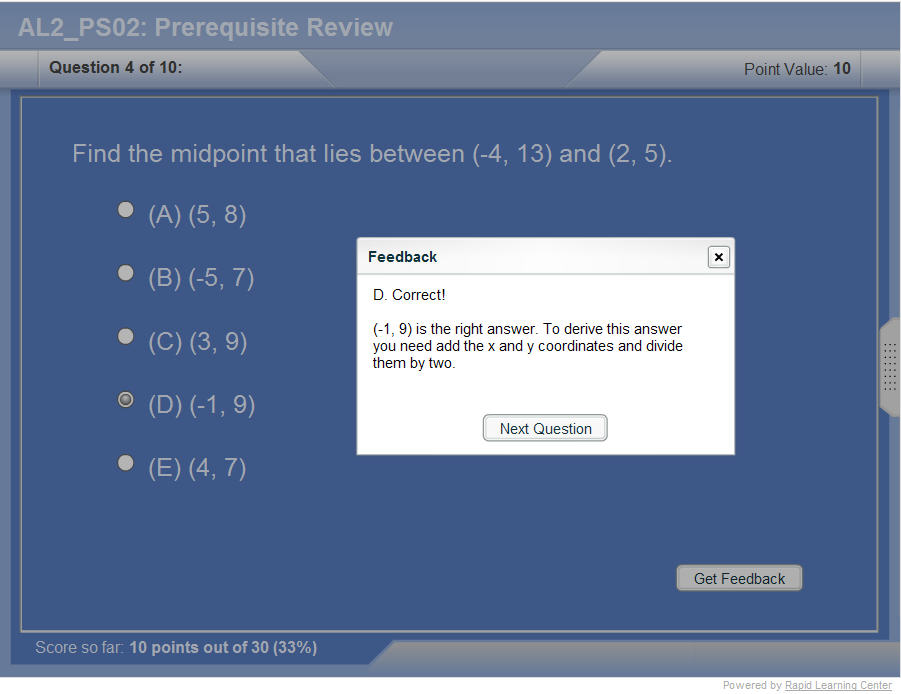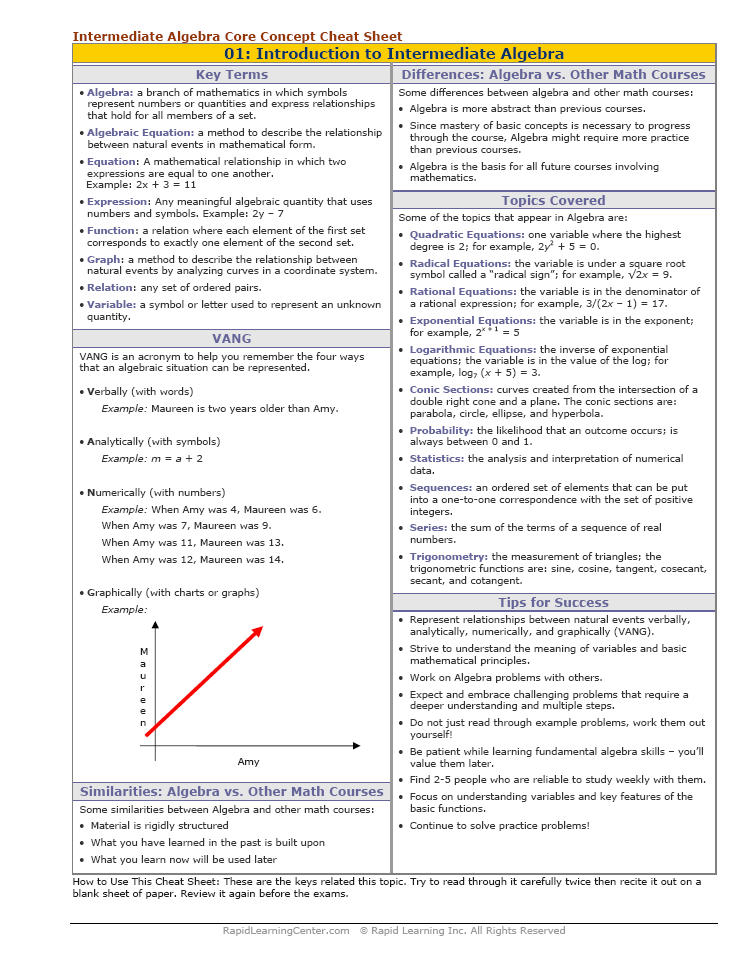How to Learn in 24 Hours?

 Need Help? M-F: 9am-5pm(PST): Toll-Free: (877) RAPID-10 or 1-877-727-4310 24/7 Online Technical Support: The Rapid Support Center vip@rapidlearningcenter.com Secure Online Order:Need Proof? Testimonials by Our Users

 Rapid Learning Courses: MCAT in 24 Hours (2021-22) USMLE in 24 Hours (Boards) Chemistry in 24 Hours Biology in 24 Hours Physics in 24 Hours Mathematics in 24 Hours Psychology in 24 Hours SAT in 24 Hours ACT in 24 Hours AP in 24 Hours CLEP in 24 Hours DAT in 24 Hours (Dental) OAT in 24 Hours (Optometry) PCAT in 24 Hours (Pharmacy) Nursing Entrance Exams Certification in 24 Hours eBook - Survival Kits Audiobooks (MP3)

 Tell-A-Friend: Have friends taking science and math courses too? Tell them about our rapid learning system.Home »  Mathematics »  Intermediate Algebra

Systems of Equations

 Topic Review on "Title": Solution of a system of equations: It is an ordered pair of numbers that is a solution of both equations in the system. Methods to solve a system of equations: Graphing, substitution, addition and matrix techniques are the methods to solve a system of equations. The point of intersection of two equations: The point in which any two equations in a system of equations meet.. System of inequalities: Multiple inequalities form a system of inequalities.

Rapid Study Kit for "Title":
 Flash Movie Flash Game Flash Card Core Concept Tutorial Problem Solving Drill Review Cheat Sheet"Title" Tutorial Summary : Multiple equations composed systems of equations. Systems of equations are solved through the use of solving methods. Solutions to systems of equations have characteristics that are shown through the use of examples. Systems of inequalities are shown in some examples with their graphs. Solving systems of inequalities have created an importance interest to individuals who want to find the most efficient way to operate a business. Systems of inequalities cannot be graph using graphing calculators due to their subtleties.

 Tutorial Features: Specific Tutorial Features: • Examples showing the different methods to solve a system of equations. • Examples with step by step assistance to solve problems. Series Features: • Concept map showing inter-connections of new concepts in this tutorial and those previously introduced. • Definition slides introduce terms as they are needed. • Visual representation of concepts • Animated examples—worked out step by step • A concise summary is given at the conclusion of the tutorial.

 "Title" Topic List: Systems and solutions of system of equationsSystem of equations and their solving methods Graphing the equations in a system of equations The graphical way to solve a system of equations Solving a system examples The addition way to solve a system of equations The substitution way to solve a system of equations Matrix techniques to solve a system of equations Solving by matrix techniquesSystems of inequalities System of inequalities and their solutions The applications of inequalities

See all 24 lessons in Intermediate Algebra, including concept tutorials, problem drills and cheat sheets:
Teach Yourself Intermediate Algebra Visually in 24 Hours